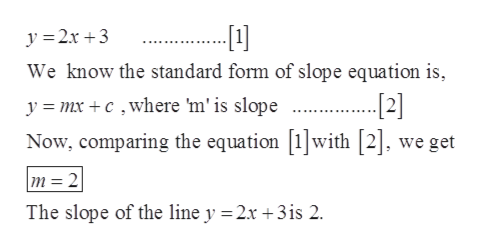# a. find the slope of the line represented by the equation y=2x +3.b. make a table of x and y values for the equation y=2x+3. how is the slope related to the table entries?

Question
1 views

a. find the slope of the line represented by the equation y=2x +3.

b. make a table of x and y values for the equation y=2x+3. how is the slope related to the table entries?

check_circle

Step 1

Part (a)

Given:help_outlineImage Transcriptionclosey =2x +3 We know the standard form of slope equation is, y = mx + c ,where 'm' is slope . Now, comparing the equation with , we get m= 2 The slope of the line y =2x +3is 2. fullscreen
Step 2

Part [b]:

For equation y = 2x +3

Put x = 0, then

Step 3

Put x = 1, ...

### Want to see the full answer?

See Solution

#### Want to see this answer and more?

Solutions are written by subject experts who are available 24/7. Questions are typically answered within 1 hour.*

See Solution
*Response times may vary by subject and question.
Tagged in
MathAlgebra

### Other